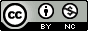## Monday, July 27, 2009

### A Primer on Logistic Regression (using Stata)

This handy introduction comes from UCLA's Statistical Computing webbook on logistic regression. If you're familiar with linear regression but you've never used logistic regression before, this is a good place to start. In addition to laying down some basic terminology and theory, this tutorial shows you how to access their example dataset, and gives you the Stata commands they use so you can follow along through their examples.

Introduction to Logistic Regression with Stata

If you're not sure when to use logistic regression, check out the two previous posts on choosing the right analysis for your data: part I and part II.